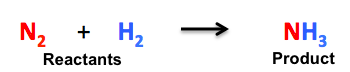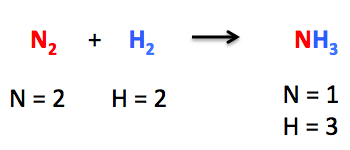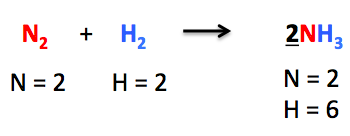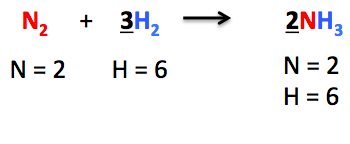Gayathri Mani

HS-PS1-2

# Equation Balancing Study Guide

Chemical equation balance represents the chemical formula of both substances that react as well as produce.

## INTRODUCTION

For any chemical equation, the number of atoms present in the reactants as well as the products should be similar. The equation represents the chemical formulas of both reacted and produced substances. Balancing the chemical equation involves the addition of substance stoichiometric coefficients to reactants and products. This is crucial because any kind of reaction must follow the law of conservation of mass alongside the law of constant proportions.

The law of conservation of mass states that the same number of atoms of each element must be present on the product side as well as the reactant side of the chemical equation.

### WHAT IS A CHEMICAL EQUATION?

A chemical reaction shows us the substances which are involved in a reaction, the reactants (substances that react), and the products (substances that are produced).

According to the law of conservation of mass, when there is a chemical reaction, the mass of the products should be equal to the mass of the reactant. Hence, the total number of atoms in the reaction does not change; therefore, for any chemical reaction, balancing the equation is vital. A balanced chemical equation occurs when the number of atoms present on both the reactant and product sides is equal.

For example,Source

In the above-mentioned chemical reaction, we can observe that nitrogen (N₂) is reacting with hydrogen (H₂) to produce ammonia (NH₃). We can see that the chemical reaction is not balanced.Source

### WHY IS THE CHEMICAL REACTION NOT BALANCED?

There are 2 nitrogen atoms and 2 hydrogen atoms on the reactant side, while on the product side, there are only 1 nitrogen atom and 3 hydrogen atoms. The number of atoms is not at all balanced on both sides. For the chemical equation to become balanced, it needs to make use of the coefficients.

### WHAT IS A COEFFICIENT?

It is a number that we place in front of any chemical formula. In the above-mentioned chemical reaction, to make the number of nitrogen atoms equal on both sides, we add 2 in front of NH₃.Source

Hence, the number of nitrogen atoms becomes equal on both sides. Then we move towards balancing the number of hydrogen atoms. We put coefficient 3 in front of H₂ so as to balance the chemical equation completely.Source

### WHAT IS A SKELETAL EQUATION IN CHEMISTRY?

When the total number of atoms of each element on both the reactant and the product side are not equal, then it could be termed as skeletal equations. In chemistry, unbalanced equations or skeletal equations play a vital role.

An example would be Mg + O₂ = 2MgO

Here, magnesium reacts with oxygen, forming magnesium oxide. Here the magnesium formula is Mg, for oxygen O₂ and for magnesium oxide is MgO.

### CONCLUSION:

• For any chemical equation, the number of atoms present in the reactants as well as the products should be similar.
• A chemical reaction shows us the substances which are involved in a reaction, the reactants, and the products.
• When the total number of atoms of each element on both the reactant and the product side are not equal, then it could be termed as skeletal equations.

### FAQs:

1. What are the three rules for balancing equations?

First, write the imbalanced equation and then calculate the number of atoms of each element on each side. Secondly, remember hydrogen or oxygen are the easiest to balance. Multiply coefficients to make the equation balanced. Thirdly, check your work to make sure the number of atoms on the reactant and product side is equal.

2. How do you practice balancing equations?

There are several balancing equation problems available on various sites and textbooks. You could do it from there using the multiply coefficient method.

We hope you enjoyed studying this lesson and learned something cool about Equation Balancing! Join our Discord community to get any questions you may have answered and to engage with other students just like you! Don't forget to download our app to experience our fun VR classrooms - we promise it makes studying much more fun 😎

## SOURCES:

1. Balanced Chemical Equation: https://study.com/academy/lesson/balanced-chemical-equation-definition-examples.html. Accessed 28 Feb 2022.
2. Balancing Chemical Equation: https://www.vedantu.com/chemistry/balancing-chemical-equations. Accessed 28 Feb 2022.